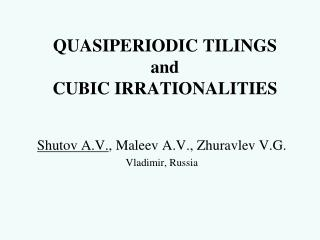# QUASIPERIODIC TILINGS and CUBIC IRRATIONALITIES - PowerPoint PPT PresentationDownload PresentationQUASIPERIODIC TILINGS and CUBIC IRRATIONALITIES

Presentation Description
Download Presentation## QUASIPERIODIC TILINGS and CUBIC IRRATIONALITIES

- - - - - - - - - - - - - - - - - - - - - - - - - - - E N D - - - - - - - - - - - - - - - - - - - - - - - - - - -
##### Presentation Transcript

1. QUASIPERIODIC TILINGS and CUBIC IRRATIONALITIES Shutov A.V., Maleev A.V., Zhuravlev V.G. Vladimir, Russia

2. Consider a cubic equation then it has a real root and two complex roots with Cubic Irrationalities with the coefficients under the conditions: We split the set Q of cubic equations with the above conditions on three sets Q3 (triangles), Q4 (squares), and Q5 (pentagons).

3. By using a greedy algorithm, any from the real integer ring Greedy Algorithms canbe decomposed in a finite series under the condition for anym>0.

4. Letζbe the real root of the equation In the case p = q = 1 (so called Rauzy case) the above order isequivalent to theconditions The Lexicographic Order of the Q3-type. Then thedigits aisatisfy a condition where means a lexicographic order. i.e. the word 111 is absent in the corresponding greedy algorithm. Similarly, in a general case, every Qk-type (k = 3; 4; 5) defines its own condition on the digits ai.

5. with thecoeficients under the corresponding Qk-restriction. The Nuclear Let Nucl = Nucl(p; q) be a set of complex numbers Then Nucl called a nuclear is a compact fractal tile.

6. Q3 equation The Nuclear

7. Q3 equation The Nuclear

8. Q4 equation The Nuclear

9. Q5 equation The Nuclear

10. Partitions of a Nuclear Each nuclear Nucl = Nucl(p; q) can be divided into small tiles respect to the first (k-1)elements a1, a1, ... , ak-1, where k is the type of an equation Qk.

11. - Green Tile - Blue Tile Partitions of a Nuclear In case - Red Tile

12. - Red Tile - Green Tile - Blue Tile Partitions of a Nuclear In case

13. Partitions of a Nuclear In case - Red Tile - Green Tile - Blue Tile - Aqua Tile

14. Partitions of a Nuclear In case - Red Tile - Red Tile - Green Tile - Green Tile - Blue Tile - Blue Tile - Aqua Tile - Aqua Tile - Yellow Tile

15. Inflations with theβ-renormalizations generate quasiperiodictilings of levels l = 0,1,2…∞

16. Inflations with theβ-renormalizations generate quasiperiodictilings of levels l = 0,1,2…∞ Level 1 2 3 4 5 6 7 8

17. The Rauzy Points The Rauzy Points Every tile includes an inner point (the Rauzy Point) which is image of the zeropointof the nuclear Nucl under some similarity. 17

18. Let R(p; q) be a set of all Rauzy points, and Rm(p, q) a set of Rauzy pointsof type m tiles. Then I(p,q) = R(p,q)'and Im(p, q) = Rm(p; q)'are correspondingparameter sets, where the dash ' is a real conjugation in the cubic field The set Im(p,q) is an intersection of the ring with some right-open interval.Moreover, the closure Im(p,q)cis the same segment, and the closure I(p,q)cis a unionof a finite number of segments. Weak Parameterization

19. Weak Parameterization

20. The case Q3: x3+x2+x=1 The case Q3: x3+x=1 The case Q4: x3 - x2+2x=1 The case Q5: x3+x2=1

21. Strong parameterization for the tiling We call the strong parameterization the partition of the parameter set into intervalswhich define the 1-crown of each tile having a parameter in the fixed interval.For this purpose we use the nuclear Nucl and the Complexity theorem.

22. The Nuclear Nucl consists of all tiles which parameters are the left ends of intervalsin the weak parameterization. The case Q3: x3+x2+x=1 The case Q3: x3+x=1 The case Q4: x3 - x2+2x=1 The case Q5: x3+x2=1

23. The Complexity Theorem Every n-crown Crnn(T) of tiles T in Til one-to-onecorresponds to tiles Tin the n-crown Crnn(Nucl) of the nuclear Nucl.Moreover, n-crowns Crnn(Ti) of all tiles Ti in Crnn(Nucl) give all types of n-crowns in the tilings.

24. The Strong Parameterization and the Partition of the Parameter Set

25. The layerwise growth of the tilings N=1 N=2 N=3 N=4 N=5 N=6 N=7 N=8

26. Growth Form Conjecture From this follows that the complexity function is asymptotically equivalent to Here is area of growth polygon. Tilings have polygonal growth forms, i.e. Moreover, with some constant c.

27. Publications • Shutov, A. V.; Maleev, A. V.; Zhuravlev, V. G. Complex quasiperiodic self-similar tilings: their parameterization, boundaries, complexity, growth and symmetry. // ActaCrystallographica Section A, 2010, 66, 427-437. • Shutov, A. V.; Maleev, A. V. Quasiperiodic plane tilings based on stepped surfaces. // ActaCrystallographica Section A, 2008, 64, 376–382. • Zhuravlev, V. G.; Maleev, A. V. Layer-By-Layer Growth of Quasi-Periodic Rauzy Tiling. // Crystallography Reports, 2007, 52, 180–186. • Zhuravlev, V. G.; Maleev, A. V. Complexity Function and Forcing in the 2D Quasi-Periodic Rauzy Tiling. // Crystallography Reports, 2007, 52, 582–588. • Zhuravlev, V. G.; Maleev, A. V. Quasi-Periods of Layer-by-Layer Growth of Rauzy Tiling. // Crystallography Reports, 2008, 53, 1–8. • Zhuravlev, V. G.; Maleev, A. V. Diffraction on the 2D Quasi-Periodic Rauzy Tiling. // Crystallography Reports, 2008, 53, 921–929. • Zhuravlev, V. G.; Maleev, A. V. Construction of 2D Quasi-Periodic Rauzy Tiling by Similarity Transformation. // Crystallography Reports, 2009, 54, 359–369. • Zhuravlev, V. G.; Maleev, A. V. Similarity Symmetry of a 2D Quasi-Periodic Rauzy Tiling. // Crystallography Reports, 2009, 54, 370–378. • Maleev, A. V.; Shutov, A. V.; Zhuravlev, V. G. 3D QuasiperiodicRauzy Tilling as a Section of 3D periodic tilling . // Crystallography Reports, 2010, 55, 427-437.

28. Thank You!